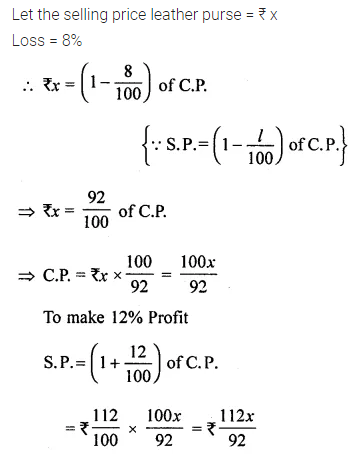# ML Aggarwal Class 8 Solutions for ICSE Maths Chapter 7 Percentage Ex 7.2

## ML Aggarwal Class 8 Solutions for ICSE Maths Chapter 7 Percentage Ex 7.2

Question 1.
Find the profit or loss percentage, when:
(i) C.P. = ₹400, S.P. = ₹468
(ii) C.P. = ₹ 13600, S.P. = ₹12104
Solution: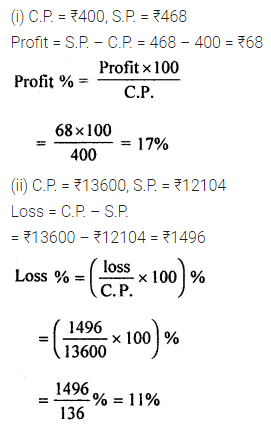Question 2.
By selling an article for ₹1636·25, a dealer gains ₹96·25. Find his gain per cent.
Solution:Question 3.
By selling an article for ₹770, a man incurs a loss of ₹110. Find his loss percentage.
Solution:Question 4.
Rashida bought 25 dozen eggs at the rate of ₹9.60 per dozen. 30 eggs were broken in the transaction and she sold the remaining eggs at one rupee each. Find her gain or loss percentage.
Solution: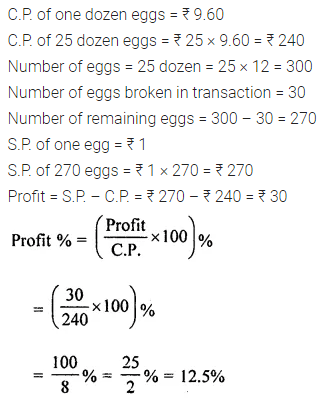Question 5.
The cost of an article was ₹20000 and ₹1400 were spent on its repairs. If it is sold for a profit of 20%, find the selling price of the article.
Solution: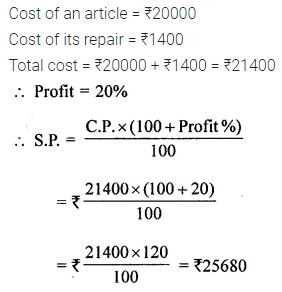Question 6.
A shopkeeper buys 200 bicycles at ₹1200 per bicycle. He spends ₹30 per bicycle on transportation. He also spends ₹4000 on advertising. Then he sells all the bicycles at ₹1350 per piece. Find his profit or loss. Also calculate it as a percentage.
Solution: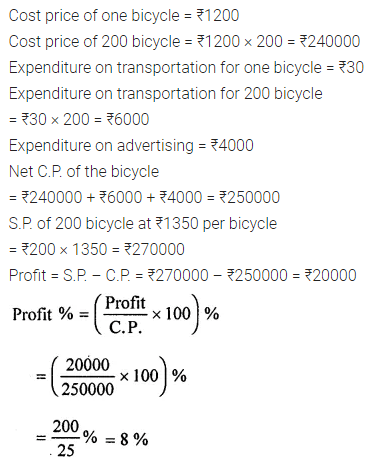Question 7.
The cost price of an article is 90% of its selling price. Find his profit percentage.
Solution: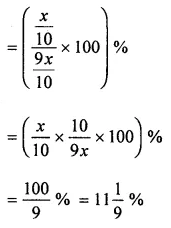Question 8.
Rao bought notebooks at the rate of 4 for ₹35 and sold them at the rate of 5 for ₹58. Calculate
(i) his gain percentage.
(ii) the number of notebooks he should sell to earn a profit of ₹171.
Solution: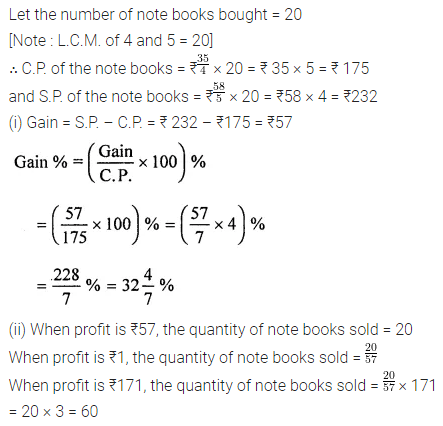Question 9.
A vendor buys bananas at 3 for a rupee and sells at 4 for a rupee. Find his profit or loss percentage.
Solution:Question 10.
A shopkeeper buys a certain number of pens. If the selling price of 5 pens is equal to the cost price of 7 Pens, find his profit or loss percentage.
Solution: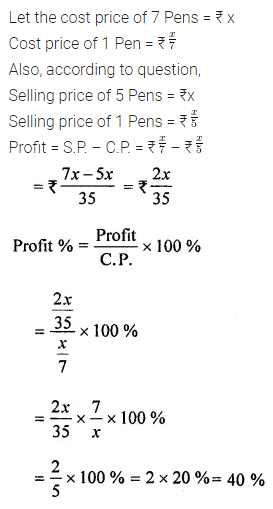Question 11.
Find the selling price, when :
(i) Cost price = ₹2360, Profit = 8%
(ii) Cost price = ₹380, Loss = 7·5%
Solution: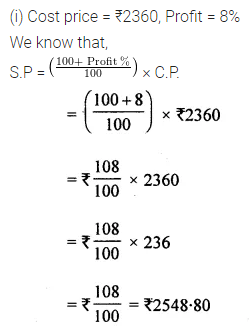Question 12.
A dealer bought a number of eggs at ₹18 a dozen and sold them at 50% profit. Find the selling price per egg.
Solution: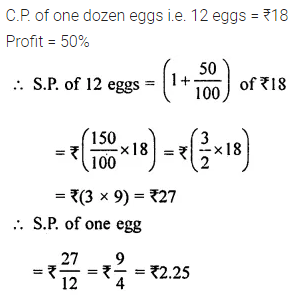Question 13.
Mr Ghosh purchased wristwatches worth ₹60000. He sold one-third of them at a profit of 30%, one-third at a profit of 20% and remaining at a loss of 5%. Calculate his overall profit or loss percentage.
Solution: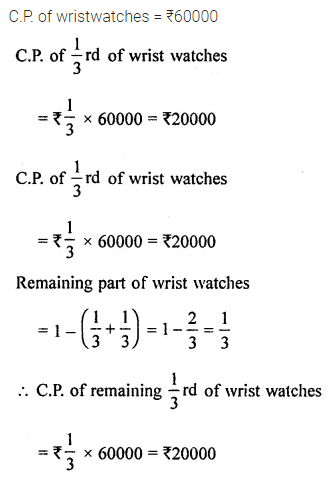Question 14.
A laptop and a mobile phone were bought for ₹40000 and ₹24000 respectively. The shopkeeper made a profit of 8% on the laptop and a loss of 12% on a mobile phone. Find his gain or loss per cent on the whole transaction.
Solution: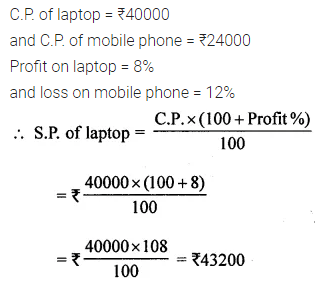Question 15.
Salman bought 40 chairs at ₹175 each fourth of them at a loss of 8%. At what price each must he sell the remaining chairs so as to gain 10% on the whole deal?
Solution: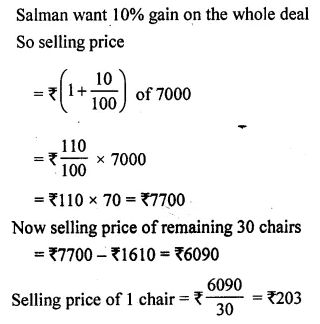Question 16.
A shopkeeper sold two electronic gadgets for ₹44000 each. The shopkeeper made a loss of 12% on one and a profit of 10% on the other. Find his overall gain or loss.
Solution:Question 17.
The manufacturing price of a T.V. set is ₹12000. The company sold it to a dealer at 20% profit and the dealer sold it to a customer at 12·5% profit. Find the price which the customer has to pay.
Solution: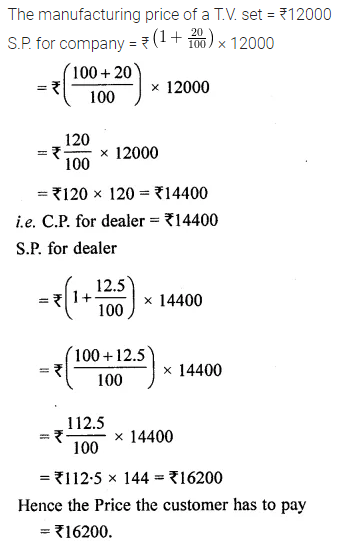Question 18.
Find the cost price, when :
(i) selling Price = ₹450, loss = 10%
(ii) selling Price = ₹690, profit = 15%
Solution:Question 19.
By selling an almirah for ₹3920, a shopkeeper would gain 12%. If it is sold for ₹4375, find his gain or loss, percentage.
Solution:Question 20.
By selling a bicycle at ₹1334, a shopkeeper would suffer a loss of 8%. At how much amount should he sell it to make a profit of $$12 \frac{1}{2}$$%?
Solution: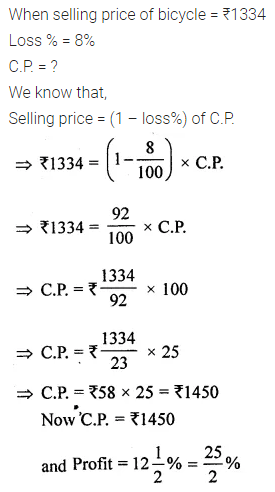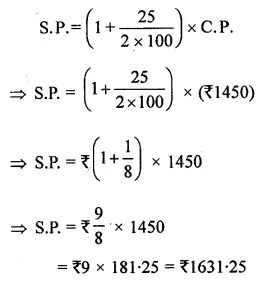Question 21.
By selling a tie for ₹252, a shopkeeper gains 5%. At what price should he sell the tie to gain 35 %?
Solution: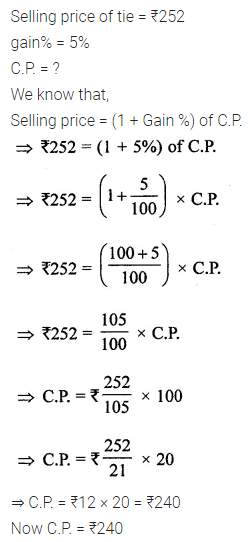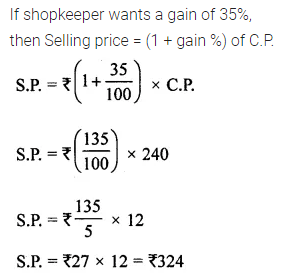Question 22.
A shopkeeper sells a bag at a 12% profit. If he had sold it for ₹39 more, he would have made 18% profit. Find the cost price of the bag for the shopkeeper.
Solution: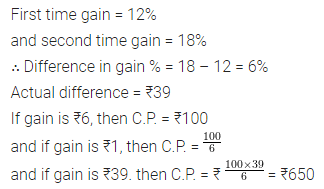Question 23.
A shopkeeper sells a sweater at a loss of 5%. If he had sold it for ₹260 more, he would have made a profit of 15%. Calculate the purchase price of the sweater.
Solution: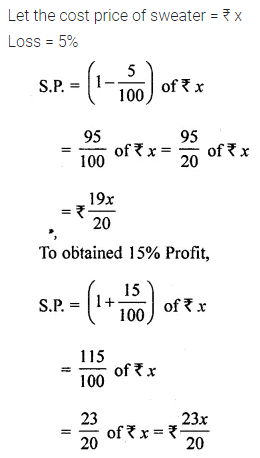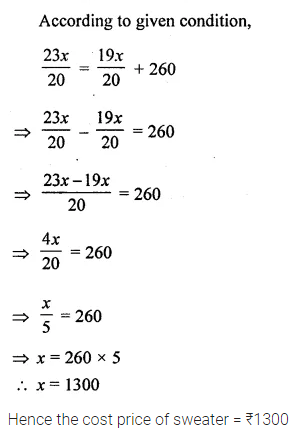Question 24.
Janki sold her leather purse at 8% loss. If she had sold it for ₹ 150 more, she would have made 12% profit. Find the selling price of the purse.
Solution: